﻿ Python: Test if circumference of two circles intersect or overlap - w3resource# Python: Test if circumference of two circles intersect or overlap

## Python Basic - 1: Exercise-45 with Solution

There are two circles C1 with radius r1, central coordinate (x1, y1) and C2 with radius r2 and central coordinate (x2, y2)

Write a Python program to test the followings -
• "C2 is in C1" if C2 is in C1
• "C1 is in C2" if C1 is in C2
• "Circumference of C1 and C2 intersect" if circumference of C1 and C2 intersect
• "C1 and C2 do not overlap" if C1 and C2 do not overlap and
• "Circumference of C1 and C2 will touch" if C1 and C2 touch

Input:
Input numbers (real numbers) are separated by a space.

Pictorial Presentation: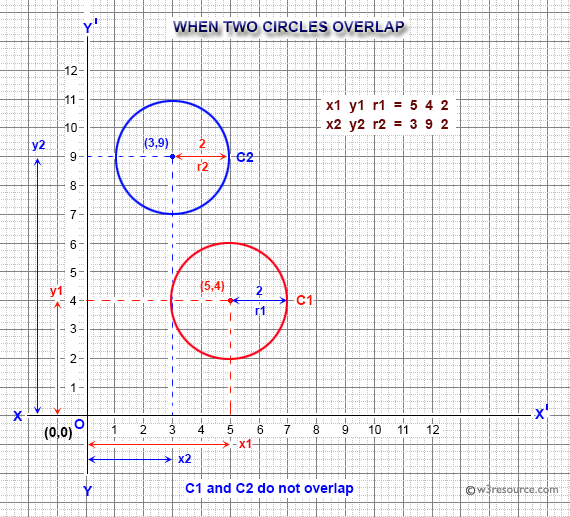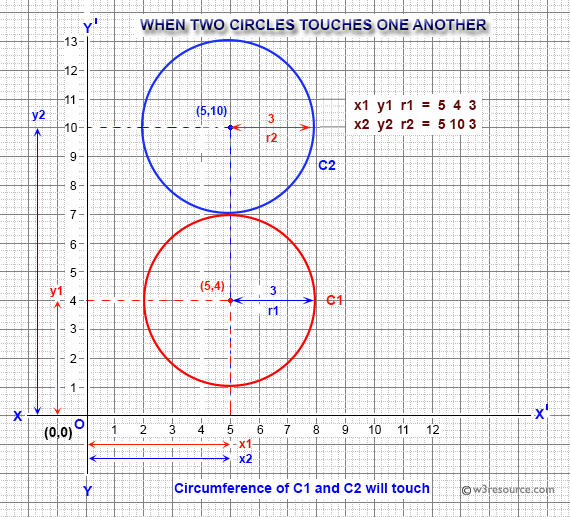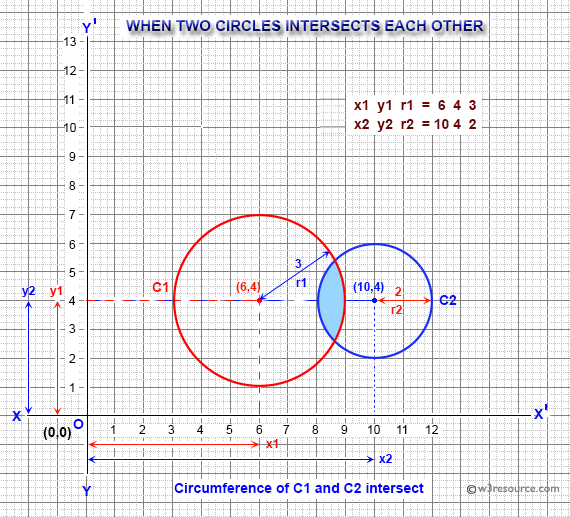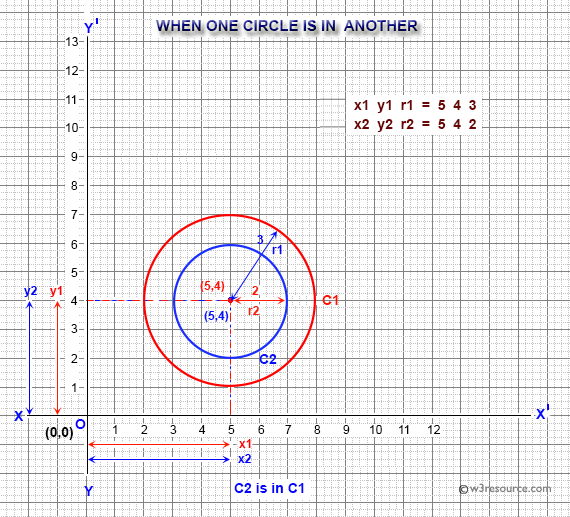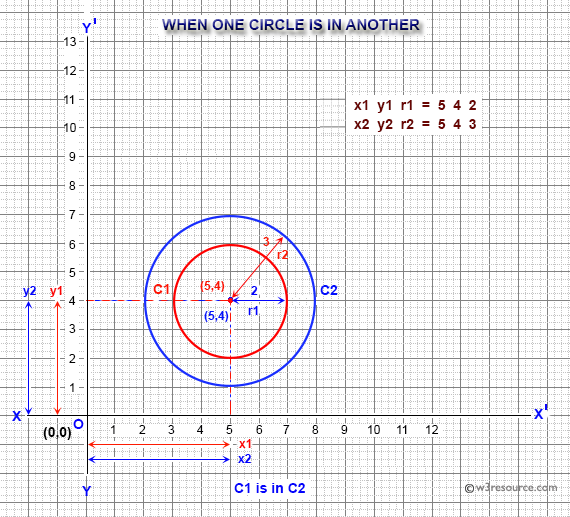Sample Solution:

Python Code:

``````import math
print("Input x1, y1, r1, x2, y2, r2:")
x1,y1,r1,x2,y2,r2 = [float(i) for i in input().split()]
d = math.sqrt((x1-x2)**2 + (y1-y2)**2)
if d <= r1-r2:
print("C2  is in C1")
elif d <= r2-r1:
print("C1  is in C2")
elif d < r1+r2:
print("Circumference of C1  and C2  intersect")
elif d == r1+r2:
print("Circumference of C1 and C2 will touch")
else:
print("C1 and C2  do not overlap")
``````

Sample Output:

```Input x1, y1, r1, x2, y2, r2:
5 4 2 3 9 2
C1 and C2  do not overlap
```
```Input x1, y1, r1, x2, y2, r2:
5 4 3 5 10 3
Circumference of C1 and C2 will touch
```
```Input x1, y1, r1, x2, y2, r2:
6 4 3 10 4 2
Circumference of C1  and C2  intersect
```
```Input x1, y1, r1, x2, y2, r2:
5 4 3 5 4 2
C2  is in C1
```
```Input x1, y1, r1, x2, y2, r2:
5 4 2 5 4 3
C1  is in C2
```

Flowchart: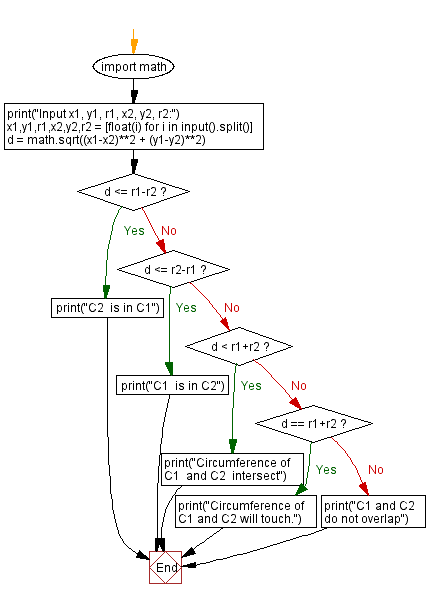Python Code Editor:

Have another way to solve this solution? Contribute your code (and comments) through Disqus.

What is the difficulty level of this exercise?

Test your Programming skills with w3resource's quiz.

﻿

## Python: Tips of the Day

How to make a flat list out of list of lists?

Given a list of lists l

```flat_list = [item for sublist in l for item in sublist]
```

which means:

```flat_list = []
for sublist in l:
for item in sublist:
flat_list.append(item)
```

is faster than the shortcuts posted so far. (l is the list to flatten.) Here is the corresponding function:

flatten = lambda l: [item for sublist in l for item in sublist]

As evidence, you can use the timeit module in the standard library:

```\$ python -mtimeit -s'l=[[1,2,3],[4,5,6], , [8,9]]*99' '[item for sublist in l for item in sublist]'
10000 loops, best of 3: 143 usec per loop
\$ python -mtimeit -s'l=[[1,2,3],[4,5,6], , [8,9]]*99' 'sum(l, [])'
1000 loops, best of 3: 969 usec per loop
\$ python -mtimeit -s'l=[[1,2,3],[4,5,6], , [8,9]]*99' 'reduce(lambda x,y: x+y,l)'
1000 loops, best of 3: 1.1 msec per loop
```

Explanation: the shortcuts based on + (including the implied use in sum) are, of necessity, O(L**2) when there are L sublists -- as the intermediate result list keeps getting longer, at each step a new intermediate result list object gets allocated, and all the items in the previous intermediate result must be copied over (as well as a few new ones added at the end). So, for simplicity and without actual loss of generality, say you have L sublists of I items each: the first I items are copied back and forth L-1 times, the second I items L-2 times, and so on; total number of copies is I times the sum of x for x from 1 to L excluded, i.e., I * (L**2)/2.

The list comprehension just generates one list, once, and copies each item over (from its original place of residence to the result list) also exactly once.

Ref: https://bit.ly/3dKsNTR